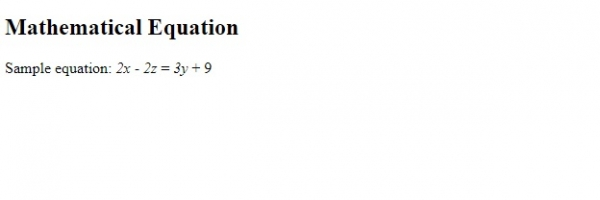# HTML <var> Tag

HTMLWeb DevelopmentFront End Technology

The <var> element in HTML is used to display mathematical expression for calculations.

Let us now see an example to implement the <var> tag in HTML -

## Example

Live Demo

<!DOCTYPE html>
<html>
<body>
<h2>Mathematical Equation</h2>
<p>Sample equation: <var>2x</var> - <var>2z</var> = <var>3y</var> + 9
</body>
</html>

## OutputIn the above example, we have the following mathematical equation -

2x – 2z = 3y – 9

The equation is represented using the <var> -

<p>Sample equation: <var>2x</var> - <var>2z</var> = <var>3y</var> + 9